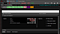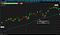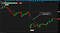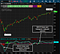# 1. How to add moving averages indicator (SMA and EMA) to the Thinkorswim platformFigure 1. This will plot the 50-period SMA on the Thinkorswim chart (see Figure 2).FIGURE 2: SPY with 50-day SMA, overall trend looks up. If the price fell below the moving average, it would be a sign of a weakening market.FIGURE 2: 10 MINUTE IN-DAY SPY CHART with 10 minute EMA in TOS. Since more recent prices carry a higher weight, the EMA tends to adjust to price changes faster than the SMA.

# 2. Moving Average Convergence Divergence (MACD to TOS)FIGURE 4: APPLICATION OF MACD in TOS. The Moving Average Convergence Divergence (MACD) indicator is plotted below the stock chart as two lines and a histogram. As the signal line (blue) crosses above and below the indicator line (yellow) and the histogram bars move above and below the zero lines, you can identify potential trend changes.